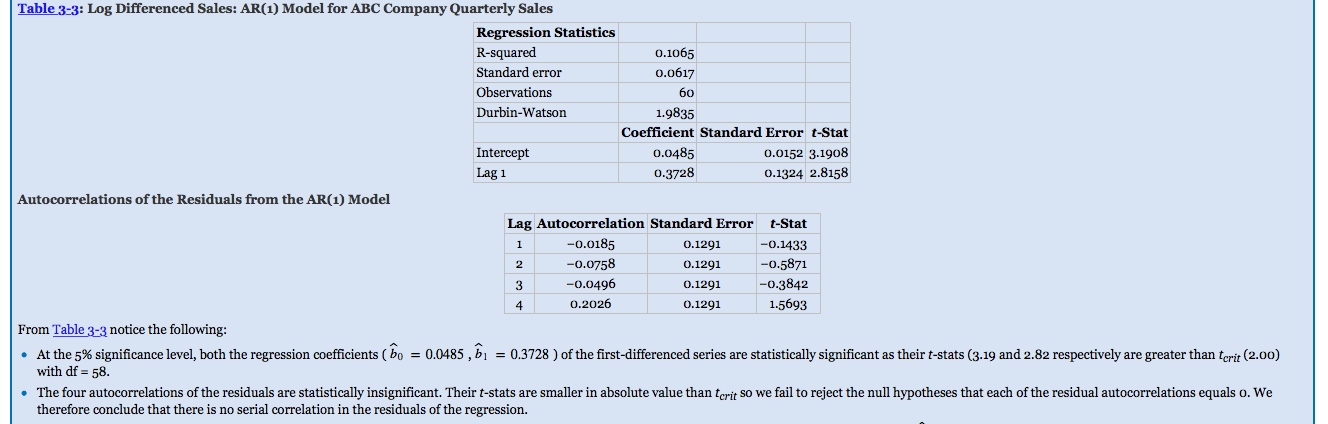CFA CFA Level 2 Confused by the term “statistically significance” based on this example

# Confused by the term “statistically significance” based on this example

• Author
Posts
• 0

Hi guys, I have some trouble understanding this example. Based on the intercept and lag 1, if the t-stat results are “statistically significant”, shouldn’t it means that the coefficients are not reliable? I am assuming that the null hypothesis for both intercepts are equal to zero and since the t-stats are significant, it means we reject the null hypothesis and conclude that the coefficients are not equal to zero? If i am right, shouldn’t this means that there is a misspecification in the model?• 4

Hi Thanks for the clarification. If the null hypothesis is not rejected for a general t-test for the regression coefficients (t-stat < t-critical), does that mean the independent variable doesn’t have the explanatory power on the model and hence it is not appropriate to use the model to draw any conclusion?

• 3

The t-test for the intercept and the lag 1 are to test for explanatory power within the regression model. For example the t-stat for lag 1 is .3728-0/.1324=2.8158 which is more than the critical t-value of 2. So they are statistically different from 0 meaning they have some form of explanatory power in the regression model.

In my opinion, I think you are mixing up the t-test for the regression coefficients versus the t-test for autocorrelation of the lagged error terms. If you are testing for autocorrelation of lagged terms then its the opposite. You want smaller values rather than larger values because you are testing correlation between term t and the kth lagged term/ (1/T^1/2). Testing to make sure the error terms are not correlated and the null is not rejected. So if correlation is small (null=0) then that means our model is correctly specified and the lagged error terms are not autocorrelated.

Hope that makes sense.

• 2

assuming simple regression with only one independent variable.

• 2

@googs1484 haha no problem! we are in the same shoes but good to have someone to discuss about this =)

• 1

Hi thank you @googs1484 for the reply! What happen if for a multi regression, the F-test is significant but on using the t-test to find out the explanatory power of each individual coefficient, at least 1 or 2 are insignificant? do you then still use the model or revamp the model by removing the coefficient which is insignificant?

• 0

@wannabe1988 correct with only 1 independent variable. Of course your model needs to specified correctly and all that jargon. With more than 1 independent variable you can use the f-test and if the f Stat is higher than the f critical value then u know at least ONE of the independent variables has explanatory power. However if u have a high a f Stat and none of the independent variables are significant then you’re looking at multicollinearity most likely.

• 0

Good question and I am not so sure of the answer to that one honestly. I am only a level II candidate 😉

However, I do not believe you would remove them. On the test, if you run into a high R squared or a significant F-test with no significant individual coefficients then you most likely have multicollinearity and should remove one of the coefficients. If only a couple are insignificant I am not sure but I know, for example, with the Beneish model which test for the probability of earnings manipulation there are two or three coefficients that are insignificant but are still included as coefficients.